Win up to 100% scholarship on Aakash BYJU'S JEE/NEET courses with ABNAT Win up to 100% scholarship on Aakash BYJU'S JEE/NEET courses with ABNAT

# JEE Main 2022 June 27 – Shift 1 Physics Question Paper with Solutions

## JEE Main 2022 27th June Morning Shift Physics Question Paper and Solutions

#### SECTION – A

Multiple Choice Questions: This section contains 20 multiple choice questions. Each question has 4 choices (1), (2), (3) and (4), out of which ONLY ONE is correct.

1. A projectile is launched at an angle ‘α’ with the horizontal with a velocity 20 ms–1. After10 s, its inclination with horizontal is ‘β’. The value of tanβ will be (g = 10 ms–2).

(A) tanα + 5secα

(B) tanα– 5secα

(C) 2tanα– 5secα

(D) 2tanα + 5secα

Sol.

$$\begin{array}{l}V_y=20\times\sin\alpha-10\times10\end{array}$$
$$\begin{array}{l}V_X=20\cos\alpha\end{array}$$
$$\begin{array}{l}\therefore \tan\beta=\frac{V_y}{V_x}=\frac{20\sin\alpha-100}{20\cos\alpha}\end{array}$$

= tanα – 5secα

2. A girl standing on road holds her umbrella at 45° with the vertical to keep the rain away. Ifshe starts running without umbrella with a speed of 15√2 kmh–1, the rain drops hit herhead vertically. The speed of rain drops with respect to the moving girl is

(A) 30 kmh–1

$$\begin{array}{l}(B)\ \frac{25}{\sqrt{2}}\textup{kmh}^{-1}\end{array}$$
$$\begin{array}{l}(C)\ \frac{30}{\sqrt{2}}\textup{kmh}^{-1}\end{array}$$

(D) 25 kmh–1

Sol.From graph,

$$\begin{array}{l}V_{RG}=15\sqrt{2}\tan45^\circ \end{array}$$
$$\begin{array}{l}=15\sqrt{2}\end{array}$$
$$\begin{array}{l}=\frac{30}{\sqrt{2}}\end{array}$$

3. A silver wire has a mass (0.6 ± 0.006) g, radius (0.5 ± 0.005) mm and length (4 ± 0.04) cm.The maximum percentage error in the measurement of its density will be

(A) 4%

(B) 3%

(C) 6%

(D) 7%

Sol.

$$\begin{array}{l}\rho=\frac{m}{V}=\frac{m}{\pi r^2\times l} \end{array}$$

∴ Percentage error in

$$\begin{array}{l}\rho=\left ( \frac{0.006}{0.6}+2\times\frac{0.005}{0.5}+\frac{0.04}{4} \right )\times100\end{array}$$

= 4%

4. A system of two blocks of masses m = 2 kg and M = 8 kg is placed on a smooth table as shown in the figure. The coefficient of static friction between two blocks is 0.5. The maximum horizontal force F that can be applied to the block of mass M so that the blocks move together will be(A) 9.8 N

(B) 39.2 N

(C) 49 N

(D) 78.4 N

Sol.amax = μg

= 0.5 × 9.8 = 4.9 m/s2

Fmax = (8 + 2) × 4.9 = 49 N

5. Two blocks of masses 10 kg and 30 kg are placed on the same straight line with coordinates(0, 0) cm and (x, 0) cm respectively. The block of 10 kg is moved on the same line through adistance of 6 cm towards the other block. The distance through which the block of 30 kgmust be moved to keep the position of centre of mass of the system unchanged is

(A) 4 cm towards the 10 kg block

(B) 2 cm away from the 10 kg block

(C) 2 cm towards the 10 kg block

(D) 4 cm away from the 10 kg block

Sol. For COM to remain unchanged,

m1x1 = m2m2

⇒ 10 × 6 = 30 × x2

⇒ x2 = 2 cm towards 10 kg block.

6. A 72 Ω galvanometer is shunted by a resistance of 8 Ω. The percentage of the total current which passes through the galvanometer is

(A) 0.1%

(B) 10%

(C) 25%

(D) 0.25%

Sol.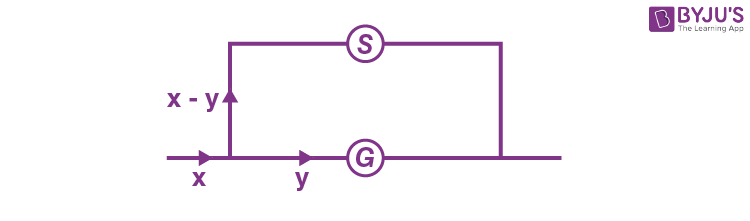From the given setup

y × RG = (x – y)(RS)

y × 72 = (x – y) × 8

⇒ 9y = xy

⇒ y = x/10 or 10% of x

Option (B)

7. Given below are two statements.

Statement-I: The law of gravitation holds good for any pair of bodies in the universe.

Statement-II: The weight of any person becomes zero when the person is at the centre of the earth.

In the light of the above statements, choose the correct answer from the options given below

(A) Both Statement I and Statement II are true

(B) Both Statement I and Statement II are false

(C) Statement I is true but Statement II is false

(D) Statement I is false but Statement II is true

Sol. Statement-I is true as law of gravitation is a universal law

Statement-II is also true as gravitational field at centre of earth is zero.

8. What percentage of kinetic energy of a moving particle is transferred to a stationary particle when it strikes the stationary particle of 5 times its mass? (Assume the collision to be head-on elastic collision)

(A) 50.0%

(B) 66.6%

(C) 55.6%

(D) 33.3%

Sol. For a head on elastic collision

$$\begin{array}{l}v_2=\frac{mu_1}{m+5m}+\frac{mu_1}{m+5m}\end{array}$$
$$\begin{array}{l}=\frac{2u_1}{6}~\textup{or}~\frac{u_1}{3}\end{array}$$

Initial kinetic energy of first mass

$$\begin{array}{l}=\frac{1}{2}mu_1^2\end{array}$$

Final kinetic energy of second mass

$$\begin{array}{l}=\frac{1}{2}\times 5m\left ( \frac{u_1}{3} \right )^2\end{array}$$
$$\begin{array}{l}=\frac{5}{9}\left ( \frac{1}{2}mu_1^2 \right )\end{array}$$

⇒ kinetic energy transferred = 55% of initial kinetic energy of first colliding mass

9. The velocity of a small ball of mass ‘m’ and density d1, when dropped in a container filled with glycerine, becomes constant after some time. If the density of glycerine is d2, then the viscous force acting on the ball, will be

$$\begin{array}{l}(A)\ mg\left ( 1-\frac{d_1}{d_2} \right )\end{array}$$
$$\begin{array}{l}(B)\ mg\left ( 1-\frac{d_2}{d_1} \right )\end{array}$$
$$\begin{array}{l}(C)\ mg\left ( \frac{d_1}{d_2}-1 \right )\end{array}$$
$$\begin{array}{l}(D)\ mg\left ( \frac{d_2}{d_1}-1 \right )\end{array}$$

Sol. Viscous force acting on the ball will be equal and opposite to net of weight and buoyant force

$$\begin{array}{l}\Rightarrow F_0=\frac{4}{3}\pi r^3d_1g-\frac{4}{3}\pi r^3d_2g\end{array}$$
$$\begin{array}{l}\frac{4}{3}\pi r^3d_1g\left ( 1-\frac{d_2}{d_1} \right )\end{array}$$
$$\begin{array}{l}=mg\left ( 1-\frac{d_2}{d_1} \right )\end{array}$$

⇒ Option (B) is correct

10. The susceptibility of a paramagnetic material is 99. The permeability of the material in Wb/A-m, is

[Permeability of free space μ0 = 4π × 10–7 Wb/A-m]

(A) 4π × 10–7

(B) 4π × 10–4

(C) 4π × 10–5

(D) 4π × 10–6

Sol. μr = x + 1

= 99 + 1 = 100

⇒ μ = μrμ0 = 100 × 4π × 10–7 Wb/Am

= 4π × 10–5 Wb/Am

⇒ Option (C) is correct

11. The current flowing through an ac circuit is given by I = 5 sin(120πt)A. How long will the current take to reach the peak value starting from zero?

$$\begin{array}{l}(A)\ \frac{1}{60}s\end{array}$$

(B) 60 s

$$\begin{array}{l}(C)\ \frac{1}{120}s\end{array}$$
$$\begin{array}{l}(D)\ \frac{1}{240}s\end{array}$$

Sol. ω = 120π

$$\begin{array}{l}\Rightarrow T=\frac{1}{60}\sec\end{array}$$

The current will take its peak value in (T/4) time

So

$$\begin{array}{l}t=\frac{T}{4}\end{array}$$
$$\begin{array}{l}=\frac{1}{240}s\end{array}$$

12. Match List-I with List-II

 List-I List-II (a) Ultraviolet rays (i) Study crystal structure (b) Microwaves (ii) Greenhouse effect (c) Infrared waves (iii) Sterilizing surgical instrument (d) X-rays (iv) Radar system

Choose the correct answer from the options given below :

(A) (a)-(iii), (b)-(iv), (c)-(ii), (d)-(i)

(B) (a)-(iii), (b)-(i), (c)-(ii), (d)-(iv)

(C) (a)-(iv), (b)-(iii), (c)-(ii), (d)-(i)

(D) (a)-(iii), (b)-(iv), (c)-(i), (d)-(ii)

Sol. UV rays are used to sterilize surgical material.Microwaves are used in radar system, infrared are used for green house effect and X-rays are used to study crystal structure.

13. An α particle and a carbon 12 atom has same kinetic energy K. The ratio of their de-Broglie wavelengths (λαC12) is :

(A) 1: √3

(B) √3 : 1

(C) 3 : 1

(D) 2 : √3

Sol. Kα = KC

$$\begin{array}{l}\frac{p_\alpha^2}{2m_\alpha}=\frac{p_c^2}{2m_c}\end{array}$$
$$\begin{array}{l}\frac{p_\alpha}{p_c}=\sqrt{\frac{m_\alpha}{m_c}}\end{array}$$
$$\begin{array}{l}\textup{So}~\frac{\lambda_\alpha}{\lambda_c}=\frac{h/p_\alpha}{h/p_c}=\sqrt{\frac{m_c}{m_\alpha}}\end{array}$$
$$\begin{array}{l}\textup{So}~\frac{\lambda_\alpha}{\lambda_c}=\sqrt{3}\end{array}$$

14. A force of 10 N acts on a charged particle placed between two plates of a charged capacitor. If one plate of capacitor is removed, then the force acting on that particle will be

(A) 5 N

(B) 10 N

(C) 20 N

(D) Zero

Sol. E between two plates is

$$\begin{array}{l}\frac{\sigma}{\varepsilon_0 }\end{array}$$
and due to one plate is
$$\begin{array}{l}\frac{\sigma}{2\varepsilon_0 }\end{array}$$
so the force will be halved.

So new force F = 5 N.

15. The displacement of simple harmonic oscillator after 3 seconds starting from its mean position is equal to half of its amplitude. The time period of harmonic motion is :

(A) 6 s

(B) 8 s

(C) 12 s

(D) 36 s

Sol. Time taken by the harmonic oscillator to move from mean position to half of amplitude is

$$\begin{array}{l}\frac{T}{12}\end{array}$$

So,

$$\begin{array}{l}\frac{T}{12}=3\end{array}$$

T= 36 sec

16. An observer moves towards a stationary source of sound with a velocity equal to one-fifth of the velocity of sound. The percentage change in the frequency will be:

(A) 20%

(B) 10%

(C) 5%

(D) 0%

Sol.

$$\begin{array}{l}f^{‘}=f_0\left [ \frac{v-v_0}{v-v_s} \right ]\end{array}$$
$$\begin{array}{l}\Rightarrow f^{‘}=f_0\left [ \frac{v+\frac{v}{5}}{v} \right ]\end{array}$$
$$\begin{array}{l}\Rightarrow f^{‘}=\frac{6f_0}{5}\end{array}$$

⇒ Percentage change = 20

17. Consider a light ray travelling in air is incident into a medium of refractive index

$$\begin{array}{l}\sqrt{2n}\end{array}$$
The incident angle is twice that of refracting angle. Then, the angle of incidence will be:

$$\begin{array}{l}(A)\ \sin^{-1}\left ( \sqrt{n} \right )\end{array}$$
$$\begin{array}{l}(B)\ \cos^{-1}\left ( \sqrt{\frac{n}{2}} \right )\end{array}$$
$$\begin{array}{l}(C)\ \sin^{-1}\left ( \sqrt{2n} \right )\end{array}$$
$$\begin{array}{l}(D)\ 2\cos^{-1}\left ( \sqrt{\frac{n}{2}} \right )\end{array}$$

Sol. According to the law,

$$\begin{array}{l}1\times\sin\theta=\sqrt{2n}\times\sin\left ( \frac{\theta}{2} \right )\end{array}$$
$$\begin{array}{l}\Rightarrow \cos\frac{\theta}{2}=\sqrt{\frac{n}{2}}\end{array}$$
$$\begin{array}{l}\Rightarrow \theta=2\cos^{-1}\left ( \sqrt{\frac{n}{2}} \right )\end{array}$$

18. A hydrogen atom in its ground state absorbs 10.2 eV of energy. The angular momentum of electron of the hydrogen atom will increase by the value of:

(Given, Planck’s constant = 6.6 × 10–34Js).

(A) 2.10 × 10–34Js

(B) 1.05 × 10–34Js

(C) 3.15 × 10–34Js

(D) 4.2 × 10–34Js

Sol.

$$\begin{array}{l}-13.6+10.2=\frac{-13.6}{n^2}\end{array}$$
$$\begin{array}{l}\Rightarrow \frac{13.6}{n^2}=3.4\end{array}$$

n = 2

$$\begin{array}{l}\Rightarrow \Delta L=2\times\frac{h}{2\lambda}-1\times\frac{h}{2\lambda}\end{array}$$
$$\begin{array}{l}=\frac{h}{2\lambda}\end{array}$$
$$\begin{array}{l} \Rightarrow \Delta L\simeq 1.05\times10^{-34}\text{Js}\end{array}$$

19. Identify the correct Logic Gate for the following output (Y) of two inputs A and B.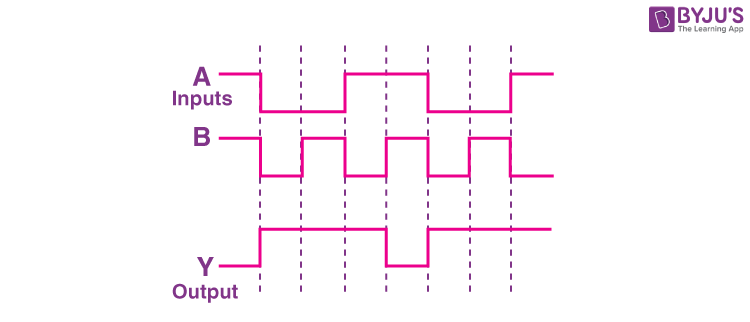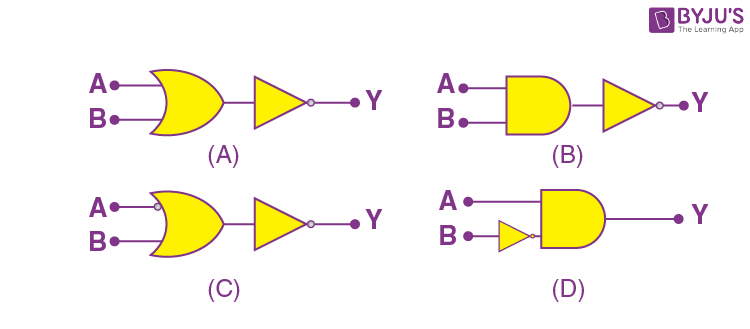Sol.

 A B Y 0 0 1 0 1 1 1 0 1 1 1 0

⇒ Y = (AB)’20. A mixture of hydrogen and oxygen has volume 2000 cm3, temperature 300 K, pressure 100 kPa and mass 0.76 g. The ratio of number of moles of hydrogen to number of moles of oxygen in the mixture will be:

[Take gas constant R = 8.3 JK–1 mol–1]
$$\begin{array}{l}(A)\ \frac{1}{3}\end{array}$$
$$\begin{array}{l}(B)\ \frac{3}{1}\end{array}$$
$$\begin{array}{l}(C)\ \frac{1}{16}\end{array}$$
$$\begin{array}{l}(D)\ \frac{16}{1}\end{array}$$

Sol. P1V = n1RT

P2V = n2RT

⇒ (100 kPa) V = (n1 + n2)RT

$$\begin{array}{l}\Rightarrow n_1+n_2=\frac{\left ( 100~kPa \right )\left ( 2000~\text{cm})^3 \right )}{8.3\times300}….(1)\end{array}$$

Also, n1 × 2 + n2 × 32 = 0.76 ….(2)

Solving (1) and (2),

n1 = 0.06

n2 = 0.02

$$\begin{array}{l}\Rightarrow \frac{n_1}{n_2}=3\end{array}$$

#### SECTION – B

Numerical Value Type Questions: This section contains 10 questions. In Section B, attempt any five questions out of 10. The answer to each question is a NUMERICAL VALUE. For each question, enter the correct numerical value (in decimal notation, truncated/rounded-off to the second decimal place; e.g. 06.25, 07.00, –00.33, –00.30, 30.27, –27.30) using the mouse and the on-screen virtual numeric keypad in the place designated to enter the answer.

1. In a carnot engine, the temperature of reservoir is 527°C and that of sink is 200 K. If the work done by the engine when it transfers heat from reservoir to sink is 12000 kJ, the quantity of heat absorbed by the engine from reservoir is ___ × 106J.

Sol.

$$\begin{array}{l}\eta=1-\frac{T_2}{T_1}\end{array}$$
$$\begin{array}{l}=1-\frac{200}{800}=\frac{3}{4}\end{array}$$
$$\begin{array}{l}\therefore \eta=\frac{W}{Q_1}\end{array}$$
$$\begin{array}{l}\Rightarrow \frac{3}{4}=\frac{12000\times10^3}{Q_1}\end{array}$$

⇒ Q1 = 16 × 106J

2. A 220 V, 50 Hz AC source is connected to a 25 V, 5 W lamp and an additional resistance R in series (as shown in figure) to run the lamp at its peak brightness, then the value of R (in ohm) will be ___.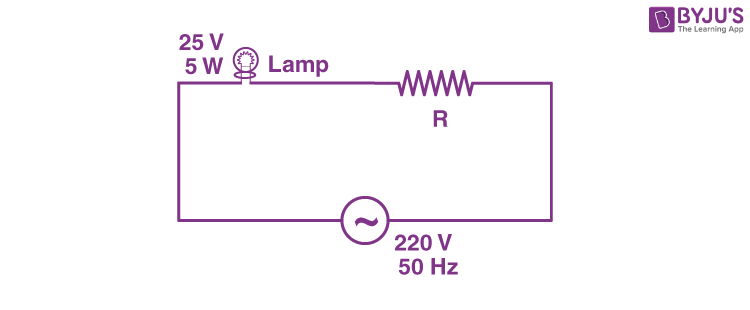Sol.

$$\begin{array}{l}R_b=\frac{\left ( 25 \right )^2}{5}=125~\Omega\end{array}$$
$$\begin{array}{l}I_\textup{rms}=\sqrt{\frac{5}{125}}=\frac{1}{5}A\end{array}$$
$$\begin{array}{l}\Rightarrow \frac{220}{R+125}=\frac{1}{5}\end{array}$$

⇒ R = 1100 – 125

= 975 Ω

3. In young’s double slit experiment the two slits are 0.6 mm distance apart. Interference pattern is observed on a screen at a distance 80 cm from the slits. The first dark fringe is observed on the screen directly opposite to one of the slits. The wavelength of light will be ___ nm.

Sol.

$$\begin{array}{l}y=\frac{d}{2},\end{array}$$
$$\begin{array}{l}\therefore \Delta x=y\frac{d}{D}\end{array}$$
$$\begin{array}{l}\Rightarrow \frac{d^2}{2D}=\frac{\lambda}{2}\end{array}$$
$$\begin{array}{l}\Rightarrow \lambda=\frac{\left ( 0.6\times 10^{-3} \right )^2}{0.8}\end{array}$$

= 450 nm

4. A beam of monochromatic light is used to excite the electron in Li++ from the first orbit to the third orbit. The wavelength of monochromatic light is found to be x × 10–10m. The value of x is ___.

[Given hc = 1242 eV nm]

Sol.

$$\begin{array}{l}E\left ( \textup{in eV} \right)=13.6\times9\left ( 1-\frac{1}{9} \right )\end{array}$$

= 13.6 × 8 eV

$$\begin{array}{l}\Rightarrow \lambda=\frac{12420}{13.6\times8}\displaystyle\overset{\circ}{\textup{A}}\end{array}$$

= 114.15 Å

5. A cell, shunted by a 8 Ω resistance, is balanced across a potentiometer wire of length 3 m. The balancing length is 2 m when the cell is shunted by 4 Ω resistance. The value of internal resistance of the cell will be ___ Ω.

Sol.$$\begin{array}{l}\frac{\varepsilon_18 }{r_1+8}3c\end{array}$$
$$\begin{array}{l}\frac{\varepsilon _14}{r_1+4}2c\end{array}$$
$$\begin{array}{l}\Rightarrow \frac{2\left ( r_1+4 \right )}{r_1+8}=\frac{3}{2}\end{array}$$

⇒ r1 = 8 Ω

6. The current density in a cylindrical wire of radius 4 mm is 4 × 106 Am–2. The current through the outer portion of the wire between radial distances

$$\begin{array}{l}\frac{R}{2}\end{array}$$
and R is _____π A.

Sol. i = A × j

$$\begin{array}{l} =\pi\left ( R^2-\frac{R^2}{4} \right )\text{j}\end{array}$$
$$\begin{array}{l}=\frac{3\pi R^2}{4}\times j\end{array}$$
$$\begin{array}{l}=\frac{3\pi\times\left ( 4\times10^{-3} \right )^2}{4}\times4\times 10^6\end{array}$$

= 48π

7. A capacitor of capacitance 50pF is charged by 100 V source. It is then connected to another uncharged identical capacitor. Electrostatic energy loss in the process is ________nJ.

Sol. Electrical energy lost

$$\begin{array}{l}=\frac{1}{2}\left ( \frac{1}{2}CV^2 \right )\end{array}$$
$$\begin{array}{l}=\frac{1}{2}\times\frac{1}{2}\times50\times10^{-12}\times\left ( 100 \right )^2\end{array}$$
$$\begin{array}{l}=\frac{500}{4} nJ\end{array}$$

= 125 nJ

8. The height of a transmitting antenna at the top of a tower is 25 m and that of receiving antenna is, 49 m. The maximum distance between them, for satisfactory communication in LOS (Line-Of-Sight) is

$$\begin{array}{l}K\sqrt{5}\times10^2m\end{array}$$
. The value of K is _______.

(Assume radius of Earth is 64×10+5 m) [Calculate upto nearest integer value]

Sol.

$$\begin{array}{l}d=\sqrt{2h_tR_e}+\sqrt{2\times h_RR_e}\end{array}$$
$$\begin{array}{l}=\sqrt{2\times25\times64\times10^5}+\sqrt{2\times49\times64\times10^5}\end{array}$$
$$\begin{array}{l}=8000\sqrt{5}+11200\sqrt{5}~m\end{array}$$
$$\begin{array}{l}=19200\sqrt{5}m\end{array}$$
$$\begin{array}{l}=192\sqrt{5}\times10^2~m\end{array}$$

9. The area of cross-section of a large tank is 0.5 m2. It has a narrow opening near the bottom having area of cross-section 1cm2. A load of 25 kg is applied on the water at the top in the tank. Neglecting the speed of water in the tank, the velocity of the water, coming out of the opening at the time when the height of water level in the tank is 40 cm above the bottom, will be ______cms–1.

[Take g=10 ms–2]

Sol. By Bernoulli’s theorem:

$$\begin{array}{l}\frac{250}{0.5}+\rho gh=\frac{1}{2}\rho v^2\end{array}$$

v = 3 m/s

v = 300 cm/s

10. A pendulum of length 2 m consists of a wooden bob of mass 50 g. A bullet of mass 75 g is fired towards the stationary bob with a speed v. The bullet emerges out of the bob with a speed v/3 and the bob just completes the vertical circle. The value of v is _______ ms–1. (if g=10 m/s2).

Sol.

$$\begin{array}{l}v_\textup{bob}=\sqrt{5gI}=\sqrt{5\times10\times2}=10~\textup{m/s}\end{array}$$

Conserving momentum:

$$\begin{array}{l}75\times v=75\times\frac{v}{3}+50\times10\end{array}$$

⇒ 50v = 50 × 10

v = 10 m/s

### Download PDF of JEE Main 2022 June 27 Shift 1 Physics Paper & Solutions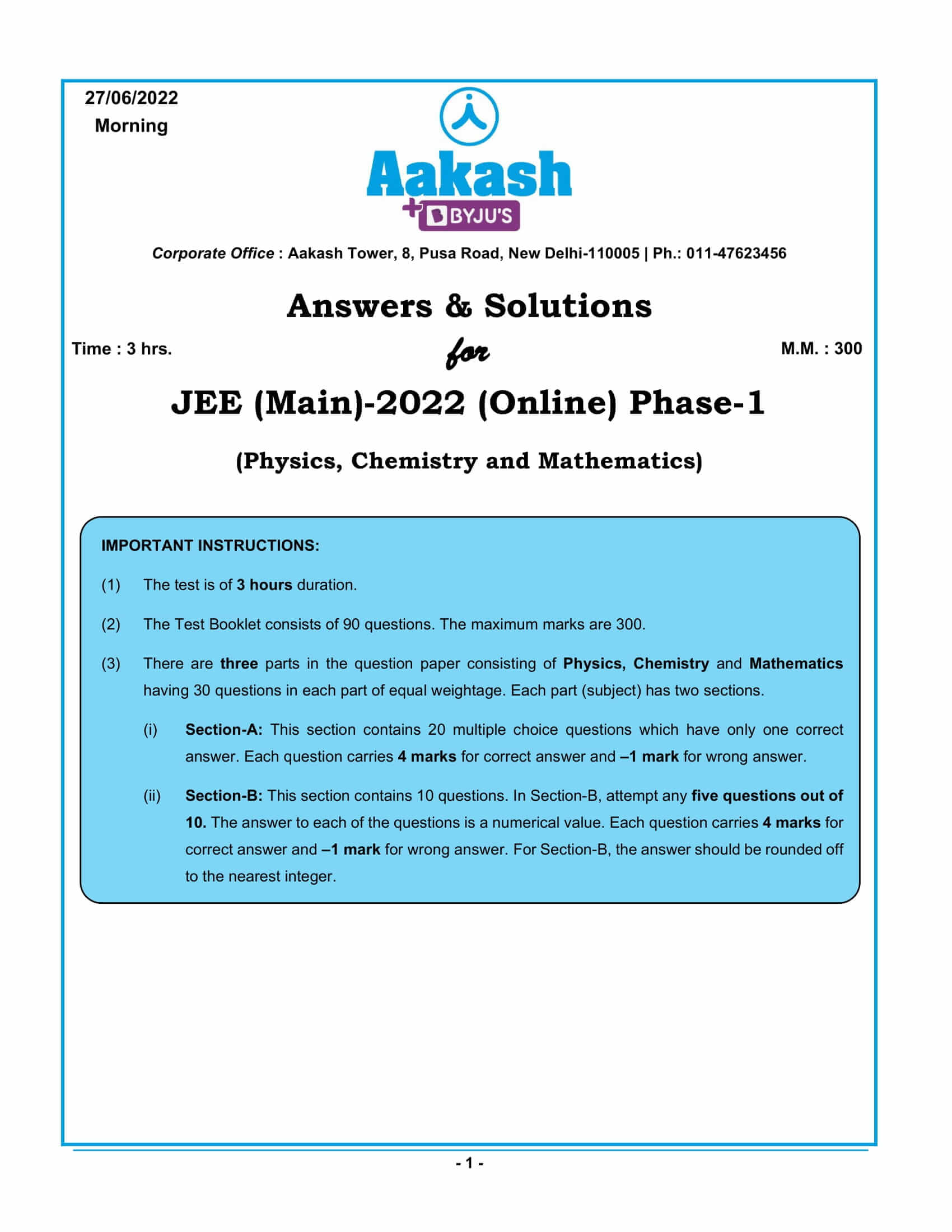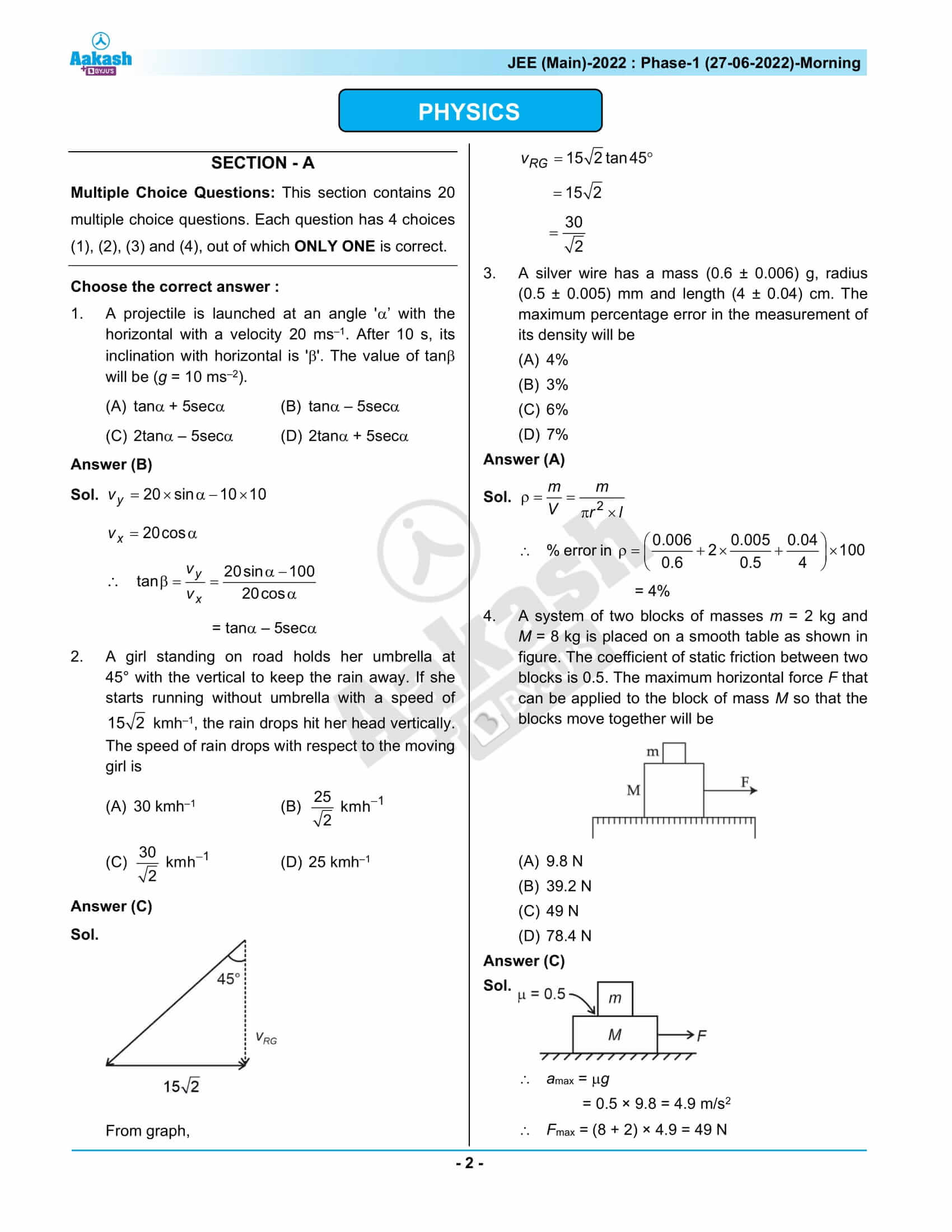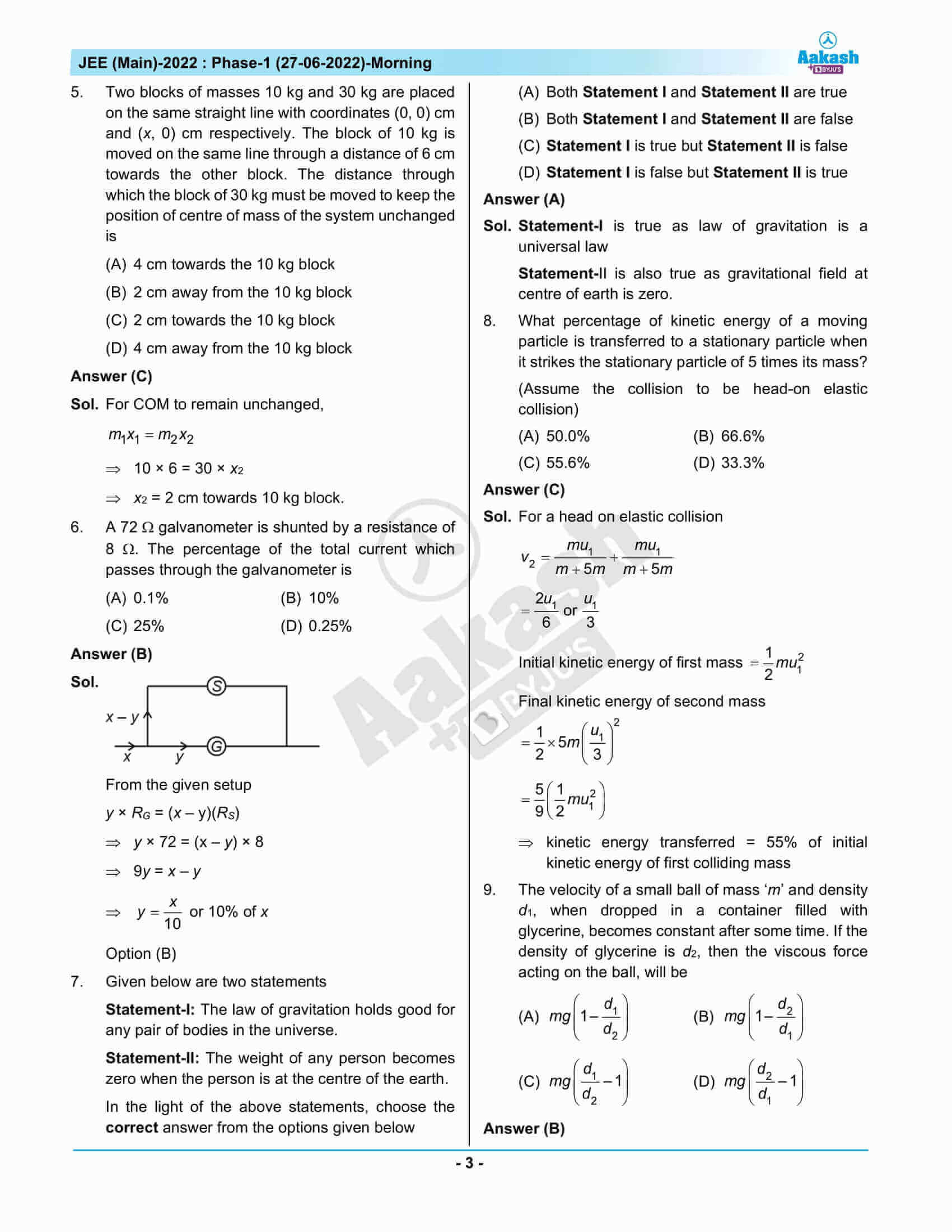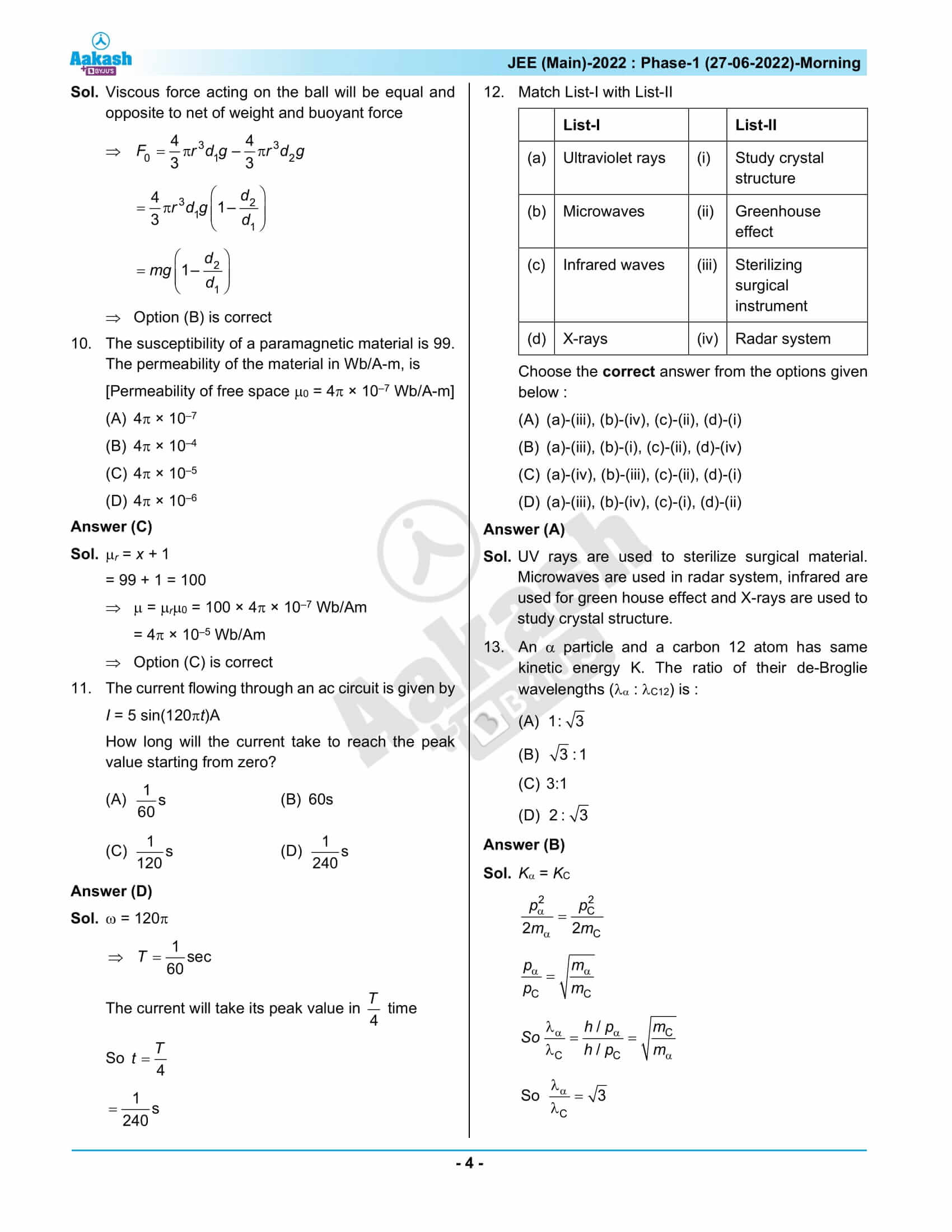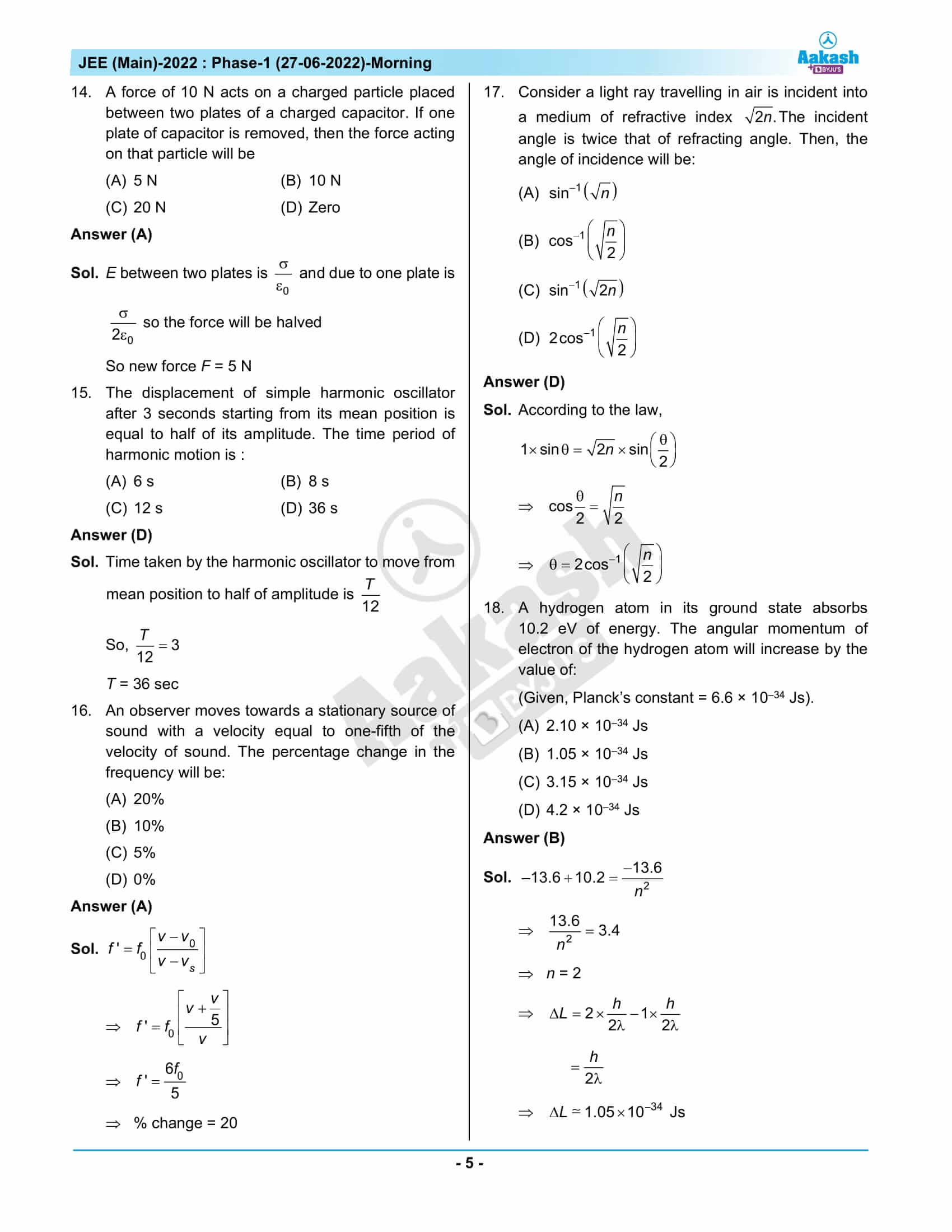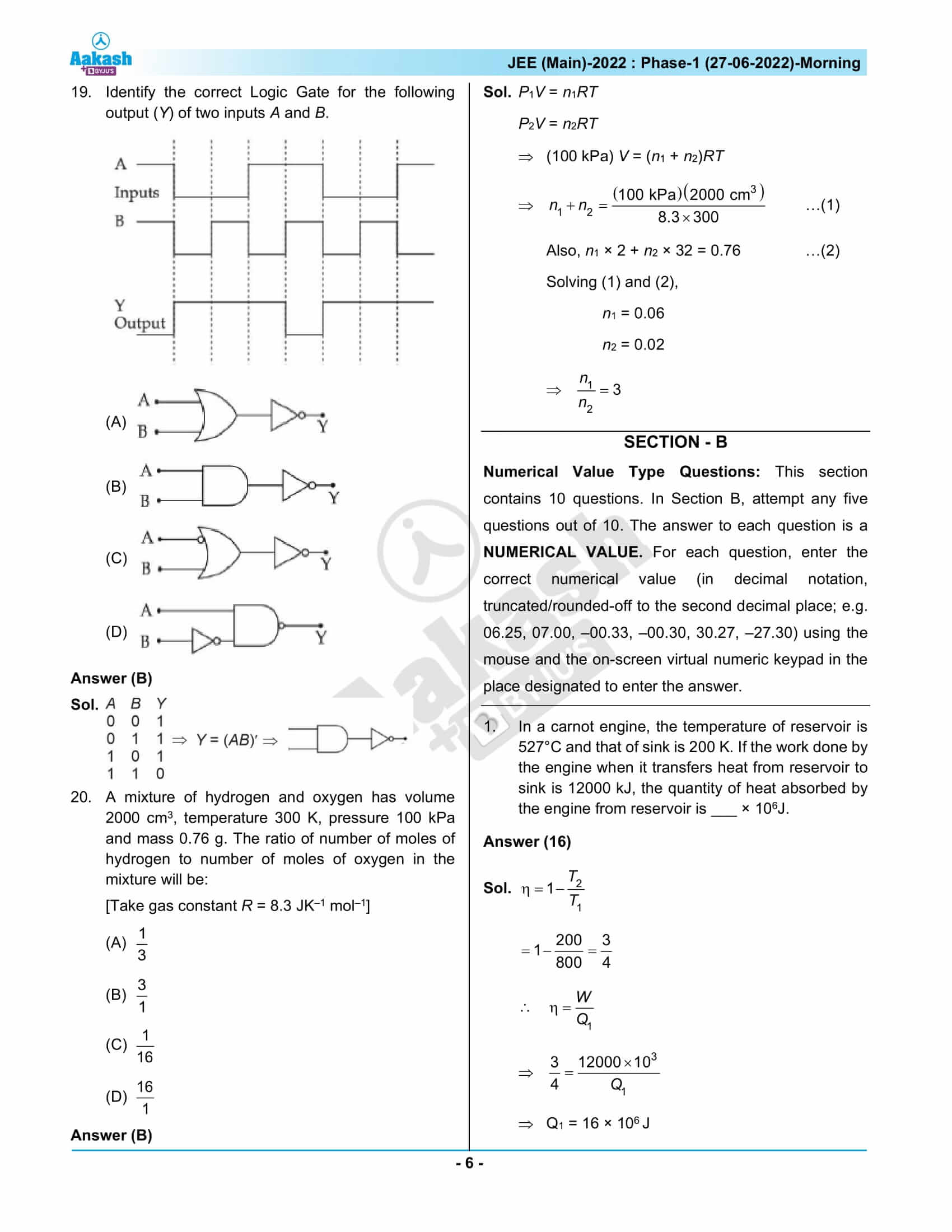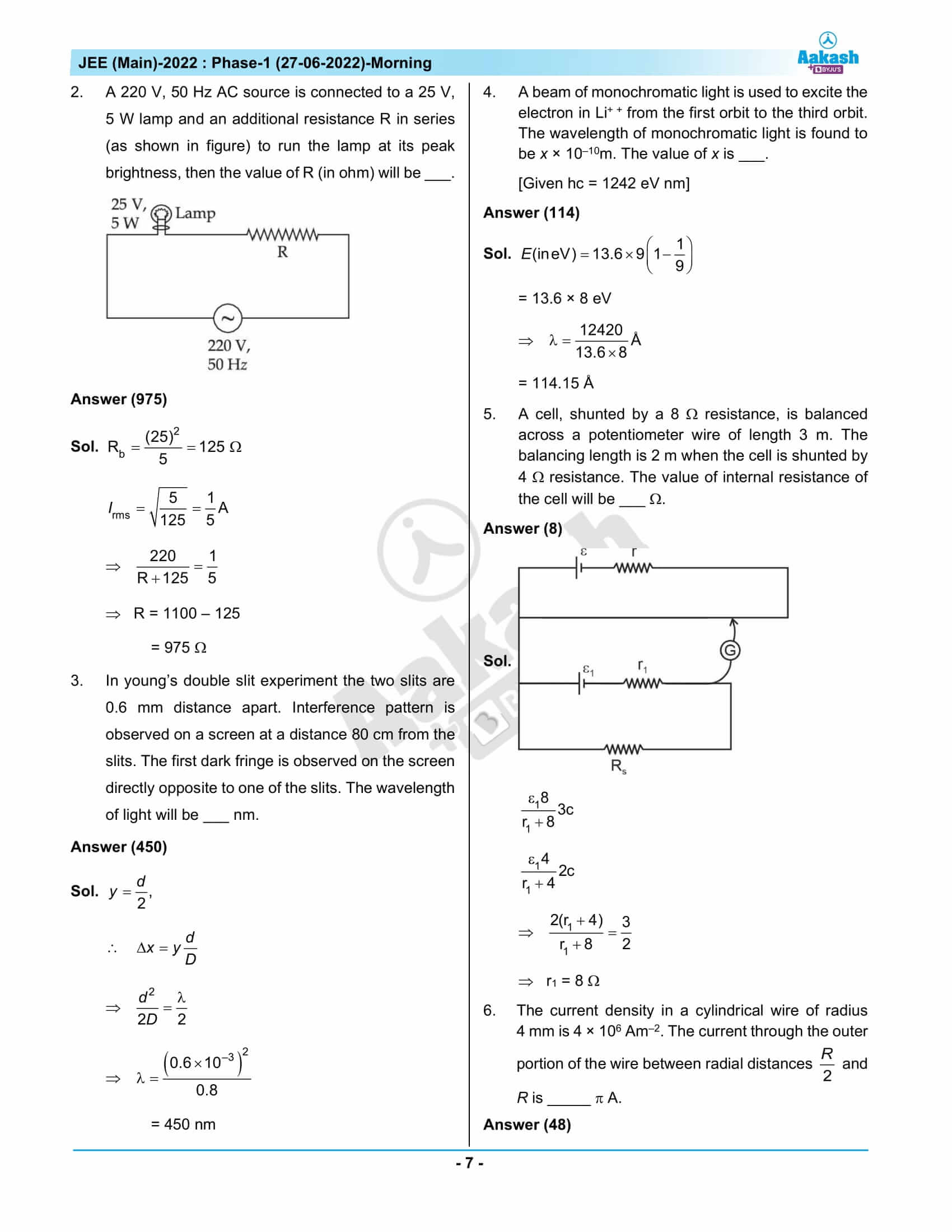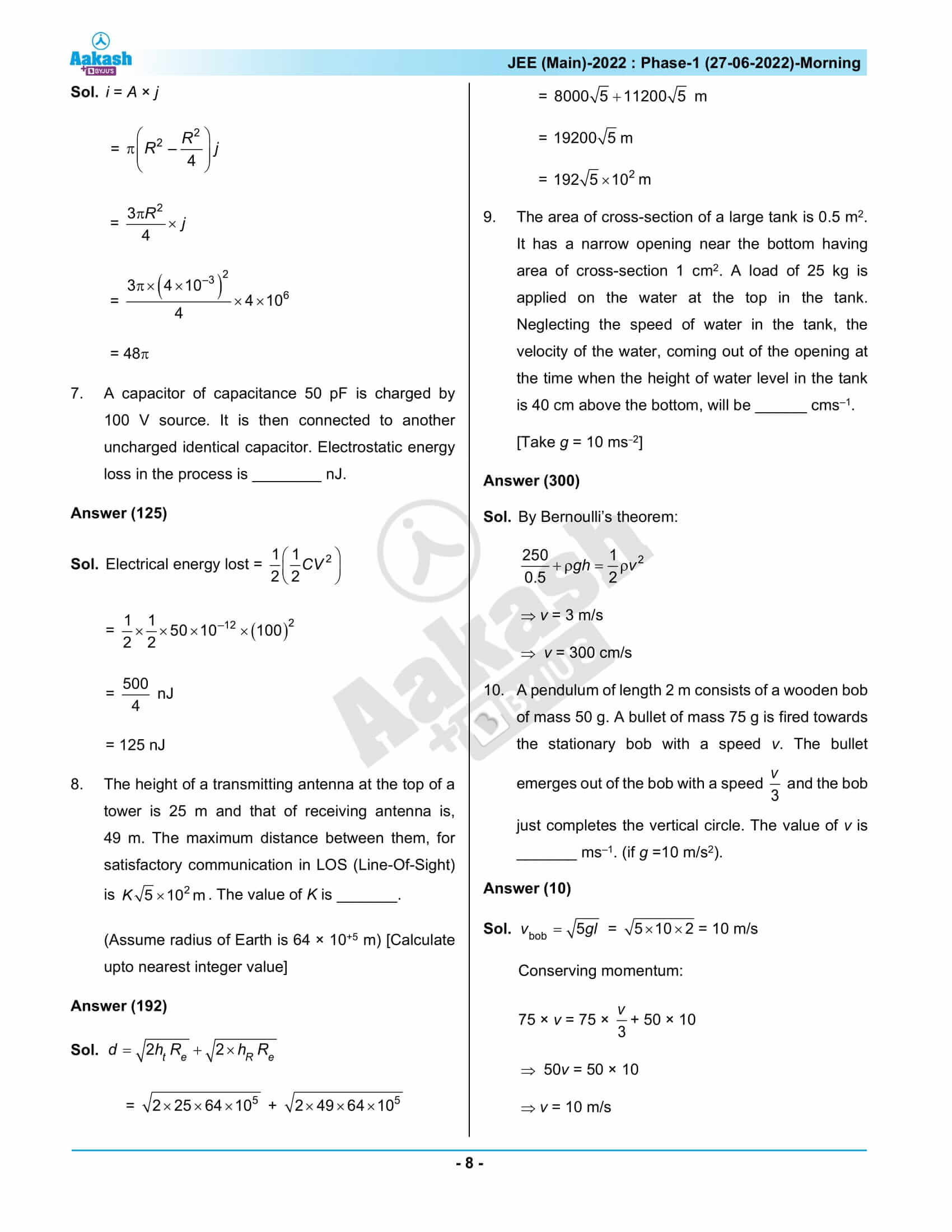## June 27th Shift 1 JEE Main 2022 Question Paper and Solutions

### JEE Main 2022 June 27 Shift 1 Question Paper – Physics Solutions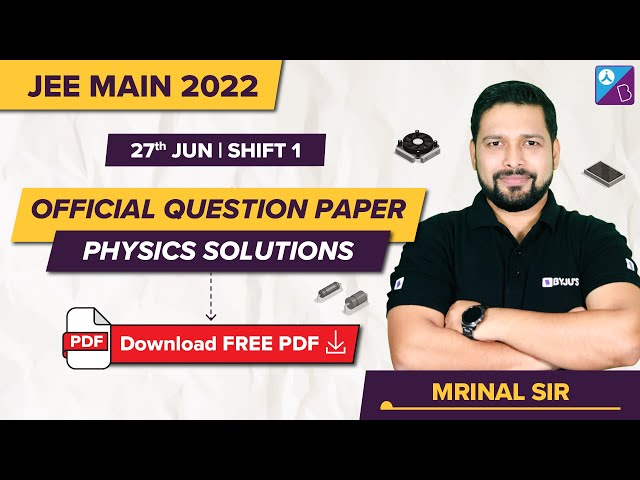### JEE Main 2022 June 27 Shift 1 Question Paper – Chemistry Solutions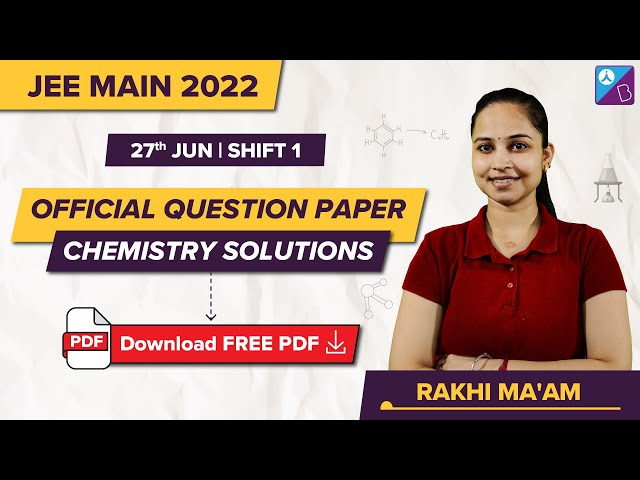### JEE Main 2022 June 27 Shift 1 Question Paper – Maths Solutions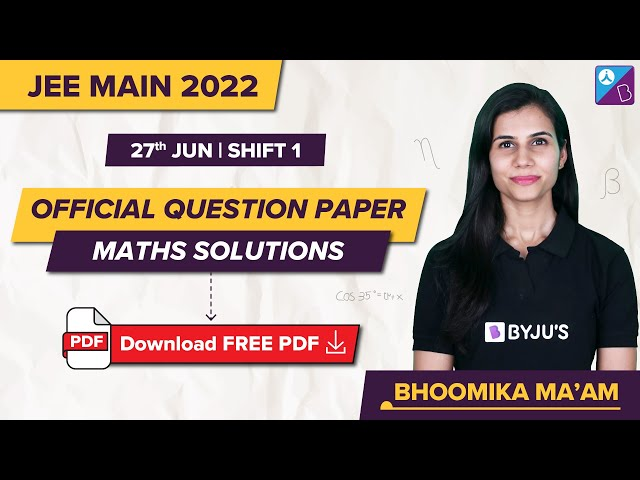## Frequently Asked Questions – FAQs

Q1

### What are the Class 11 Physics chapters included in JEE Main 2022 June 27 shift 1 Physics question paper?

The chapters included are:
Laws of Motion
Units and Measurements
System of Particles and Rotational Motion
Motion In A Plane
Work, Energy and Power
Gravitation
Oscillations
Waves
Thermodynamics
Mechanical Properties of Fluids

Q2

### What are the Physics chapters included from Class 12 in JEE Main 2022 27th June morning shift Physics question paper?

The Physics chapters included from Class 12 in JEE Main 2022 27th June morning shift Physics question paper are:
Electromagnetic Waves
Current Electricity
Electrostatic Potential and Capacitance
Dual Nature of Radiation and Matter
Semiconductor Electronics: Materials, Devices and Simple Circuits
Work, Energy and Power
Ray Optics and Optical Instruments
Wave Optics
Magnetism and Matter
Alternating Current
Moving Charges and Magnetism
Atoms
Communication Systems
Electrostatic Potential and Capacitance

Q3

### What was the topic from which question was asked in the chapter Motion in a Plane?

The topic from which question was asked in the chapter Motion in a Plane was the Rain and Man problem.

Q4

### What was the difficulty level of the Physics questions asked in JEE Main 2022 June 27 shift 1 Physics paper?

Most of the Physics questions were either easy or of moderate difficulty and few were difficult.

Q5

### What was the overall difficulty level of the JEE Main 2022 question paper 27th June shift 1?

The overall difficulty level of the JEE Main 2022 June 27 morning shift question paper is 1.73 out of 3.